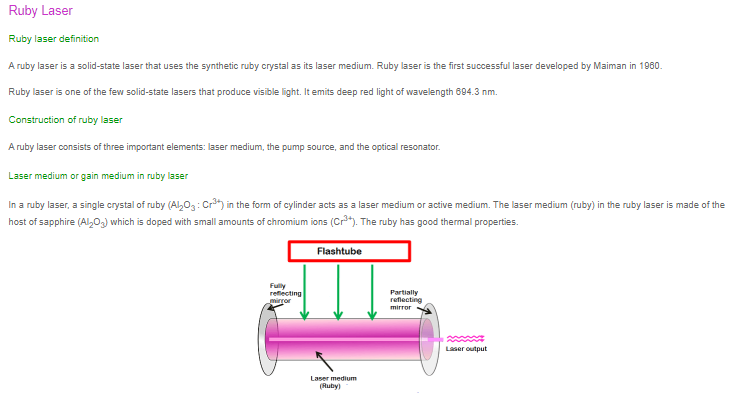×#### Thank you for registering.

One of our academic counsellors will contact you within 1 working day.

Click to Chat

1800-1023-196

+91-120-4616500

CART 0

• 0

MY CART (5)

Use Coupon: CART20 and get 20% off on all online Study Material

ITEM
DETAILS
MRP
DISCOUNT
FINAL PRICE
Total Price: Rs.

There are no items in this cart.
Continue Shopping
```
From points on the circle x 2 + y 2 = a 2 tangents are drawn to hyperbola x 2 -y 2 =a 2 . Prove that locus of middle points of chords of contact is the curve (x 2 -y 2 ) 2 = a 2 (x 2 +y 2 ).
From points on the circle x2 + y2 = a2 tangents are drawn to hyperbola x2-y2=a2. Prove that locus of middle points of chords of contact is the curve (x2-y2)2 = a2 (x2+y2).

```
3 years agoSaurabh Koranglekar
10341 Points
``````
9 months ago
```							Let a point P(x1, y1) be on the circle C1: x²+ y² = a².       --- (1)Let the tangents to the Hyperbola H1: x² - y² = a²  ---- (2), from P be PQ and PR, touching H1 at Q(x2, y2) and R(x3, y3).   So PQ:  x *x2 - y * y2 = a², and  PR : x * x3 - y * y3 = a²       --- (3) As PQ and PQ pass through P,        x1 * x2 - y1* y2 = a²  and   x1 * x3 - y1 * y3 = a²      --- (4)Equation of QR - Chord of Contact containing Q & R - is clearly,           x1 * x - y1 * y = a².    --- (5)Midpoint of chord of contact QR is: S(α, β) = [ (x2+x3)/2, (y2 +y3)/2 ].Adding two equations in (4), we get  x1 α - y1 β = a²    --- (6)Equation of chord of contact QR of H1 with its mid point at S(α, β) is given by formula:                                  T    =   S1    --- (7)            ie., x α - β y - a²  =  α² - β² - a²           =>  x α - y β = α² - β².      --- (8)Equations (5) and (8) represent the same Chord of contact QR:    So   x1 / α = y1 / β = a²/(α² - β²)     or   x1 = α a²/(α²+β²)   and   y1 = β a²/(α² - β²)      --- (9)Substitute (9) in eq (1) to get :         (a⁴ α² + a⁴ β² )/(α² - β²)² = a²Replace S(α, β) by (x,y) to get the locus.       =>  (x² + y²) a² = (x² - y²)²
```
9 months ago
Think You Can Provide A Better Answer ?

## Other Related Questions on Analytical Geometry

View all Questions »### Course Features

• 731 Video Lectures
• Revision Notes
• Previous Year Papers
• Mind Map
• Study Planner
• NCERT Solutions
• Discussion Forum
• Test paper with Video Solution### Course Features

• 53 Video Lectures
• Revision Notes
• Test paper with Video Solution
• Mind Map
• Study Planner
• NCERT Solutions
• Discussion Forum
• Previous Year Exam Questions